# Division Worksheets for year 4

Get ready to dole out the division! These 15 worksheets feature different maths challenges to keep your maths student busy.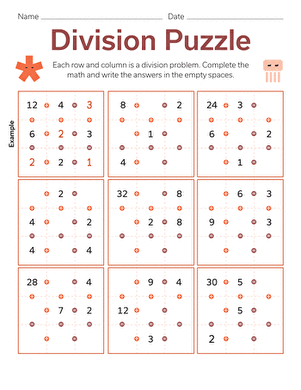### Division Puzzle

Don't be square, have a little fun with division! Each row and column is a division problem. Can you fill in the blanks?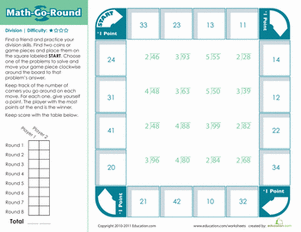### Maths-Go-Round: Division (Easy)

Have your child grab a friend and take on this board game that makes difficult division problems easy and fun.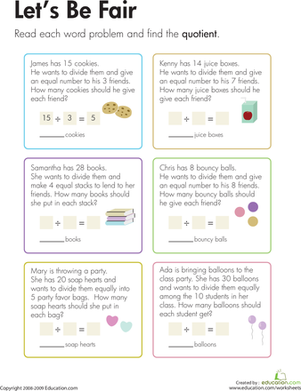### Division: Let's Be Fair!

The characters in these word problems want to share their items, equally, among friends. Kids divide to solve the problems on this year four maths worksheet.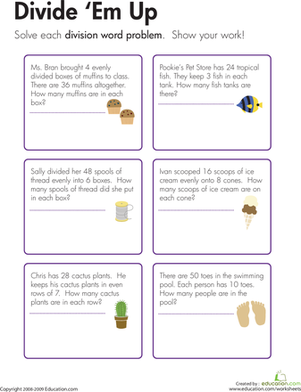### Division Word Problems: Divide 'Em Up!

Kids use details from word problems on this year four maths worksheet to construct and solve division problems in which a multidigit number is evenly divided.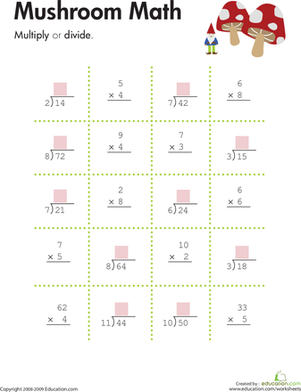### Multiplication & Division: Mushroom maths

Kids multiply or divide to complete each equation on this year four maths worksheet.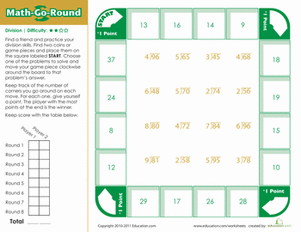### Maths-Go-Round: Division (Medium)

If your division aficionado wants to show off his maths skills, give him our long division board game. He'll play against a friend to compete for the best score.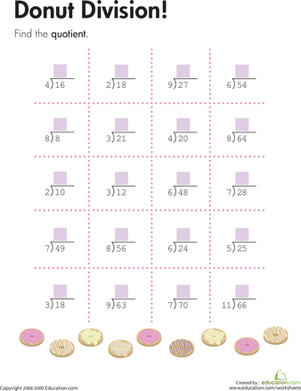### Donut Division Problems!

Do some donut division with this maths worksheet. Kids will solve division problems alongside a batch of tasty donuts.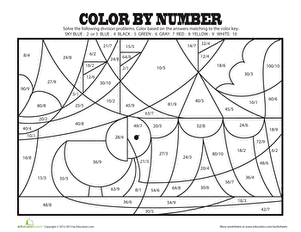### Circus colour By Number

Find what is hidden in this colour by number worksheet! colour-coding answers can help your child practise and memorize division facts and practise creativity.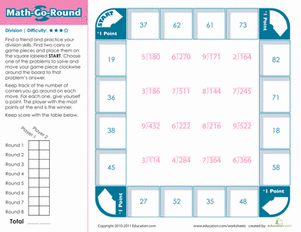### Maths-Go-Round: Division (Hard)

Kids with good long division skills will enjoy playing this mathematical board game. Your child and a friend will use maths to earn points and win.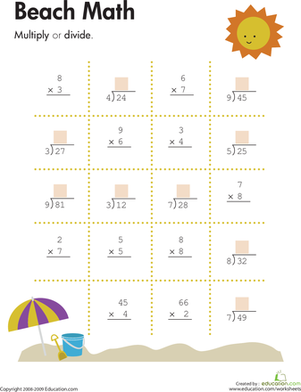### Multiplication & Division: Beach maths

Kids multiply or divide to complete each equation on this year four maths worksheet.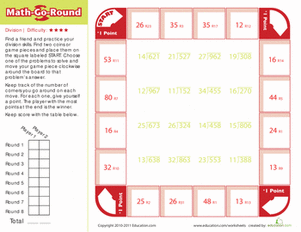### Maths-Go-Round: Division (Expert)

Challenge your child with this long division board game. He'll solve equations to earn points and win the game.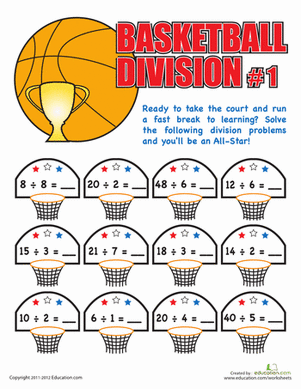Is your child a times tables all-star? Give her a fun challenge with a few division problems, a great way to review the times tables.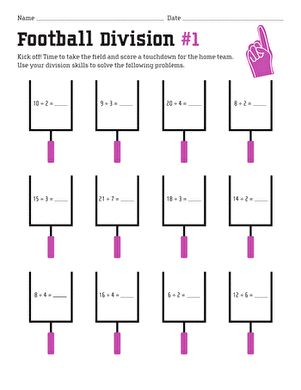### Football Division #1

Children tackle division problems with this football-themed worksheet.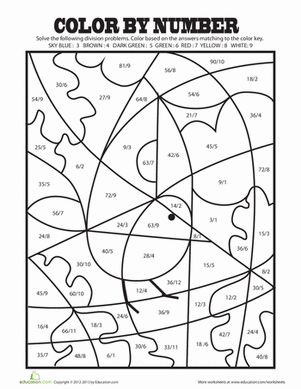### Colour By Number Division

Challenge your third grader with some basic division problems and then use his answers to complete a colour-by-number division masterpiece!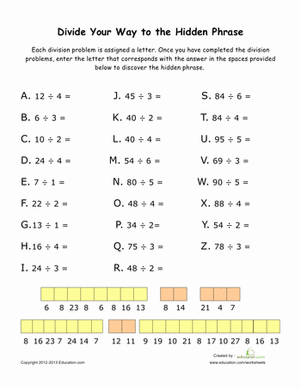### Fun With Division

Make maths practise fun with this division sheet! As she solves the problems, your child will uncover a hidden phrase at the bottom.

Create new collection

0

### New Collection>

0Items

What could we do to improve Education.com?